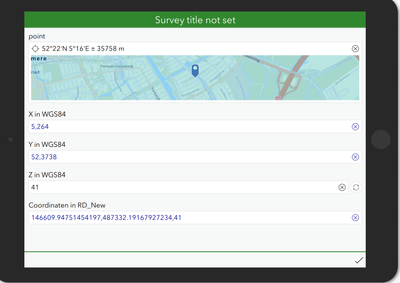# Transform Z-height (WGS84) to Local system (RD-New + NAP)

218
0
10-28-2022 03:52 AMNew Contributor II

Hello!,

I'm working with pro4js to transform xyz coordinates. In index.js (via WebpackJS) i build the following javascript function:

``````export function reprojectToRDNewNAP(x,y,z) {
try {
if (isNaN(x) || isNaN(y)) {
Error("Please provide numbers for x and/or y")
}

if (!isFinite(x) || !isFinite(y) | !isFinite(z)) {
Error("Please provide finite numbers for x, and/or y")
}

const projectXYTo = "+proj=sterea +lat_0=52.1561605555556 +lon_0=5.38763888888889 +k=0.9999079 +x_0=155000 +y_0=463000 +ellps=bessel +units=m +towgs84=565.2369,50.0087,465.658,-0.406857330322398,0.350732676542563,-1.8703473836068,4.0812 +no_defs +type=crs";
proj4.defs('RD_NAP', projectTo)
let xyz = proj4('RD_NAP', [x,y,z]);
}
catch (e) {
return "No value returned"
}
}``````

After building (npm run-script build) and placing the constructed JavaScript file in the survey123 scrips folder, i get the following results:While x and y are correctly transformed, nothing is done with the z coordinates.

Is there a way to transform the z-coordinate too?

XLSForm is in the attachment

Thanks!

Bart Franken

Tags (3)
0 Replies